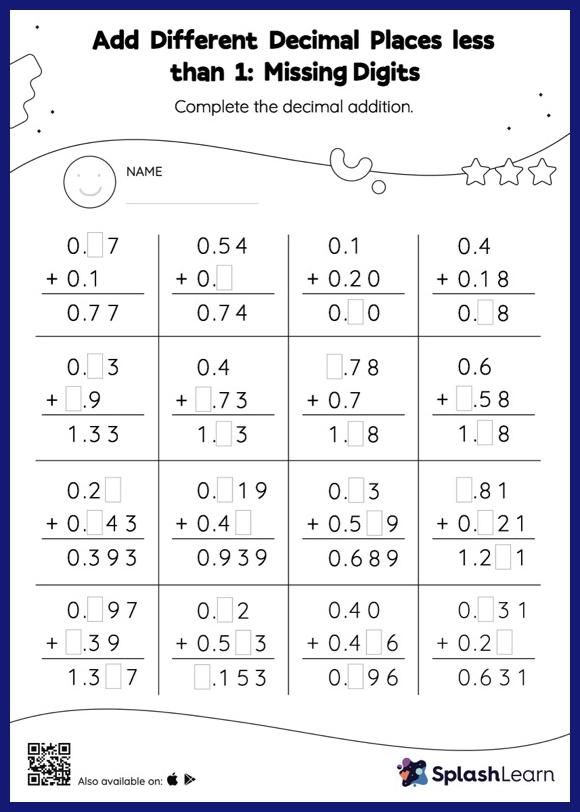# Add Different Decimal Places less than 1: Missing Digits Worksheet

Home > Add Different Decimal Places less than 1: Missing DigitsAdd different decimal places less than 1 worksheet is a great way to help learners become fluent with the concept of addition. When adding decimals on this worksheet, students align the decimal points and use zero as a placeholder. Then to find the missing number in the add different decimal places less than 1 worksheet, students then apply the relationship between addition and subtraction. In this worksheet, numbers are laid one on top of another (vertical format). This encourages students to use the inherent place value structure to solve the problems.